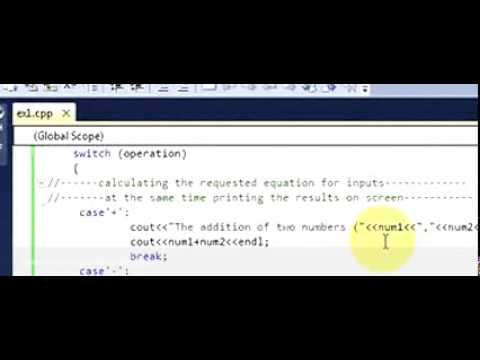# C++ program to Make Simple calculator

### Write a C++ program to MAke Simple calculator

C++ Exercise 1:
Write a program and call it calc.cpp which is the basic calculator and receives three values from input via keyboard.
 in this C++ tutorial The first value as an operator (Op1) should be a char type and one of (+, -, *, /, s) characters with the following meanings:
o ‘+’ for addition (num1 + num2)
o ‘-’ for subtraction (num1 - num2)
o ‘*’ for multiplication (num1 * num2)
o ‘/’ for division (num1 / num2)
o ‘s’ for swap
in this C++ tutorial Program should receive another two operands (Num1, Num2) which could be float or integer.
 in this C++ tutorial The program should apply the first given operator (Op1) into the operands (Num1, Num2) and prints the relevant results with related messages in the screen.
 in this C++ tutorial Swap operator exchanges the content (swap) of two variables, for this task you are not allowed to use any further variables (You should use just two variables to swap).

```#include<iostream>
#include<cmath>

using namespace std;

int main()
{
//-------defining variables and initializing them-------------
double num1,num2;
char operation,redo;
//--------Printing my name on screen----------------
cout<<"Welcome to the calculater program v.1.0 written by Your Name"<<endl;
cout<<"***************************************************************"<<endl;
cout<<endl<<endl<<endl;
//--here do loop is used so that the program can be used more then one time
//without exiting the run screen---------------------------
do
{
//----receiving the variables from input--------------
cout<<" Please enter an operation which you like to calculate (+,-,*,/,s)";
cout<<"[s stands for swap]:";
cin>>operation ;
cout<<endl<<endl;
cout<<" Please enter two numbers to apply your requested operation(";
cout<<operation<<"):"<<endl<<"1st num:";
cin>>num1;
cout<<"2nd num:" ;
cin>>num2;
cout<<endl;
//---used switch function so thet the operater can be decided------------
switch (operation)
{
//------calculating the requested equation for inputs-------------
//-------at the same time printing the results on screen-----------
case'+':
cout<<"The addition of two numbers ("<<num1<<","<<num2<<"):";
cout<<num1+num2<<endl;
break;
case'-':
cout<<"The substraction of two numbers ("<<num1<<","<<num2<<"):";
cout<<num1-num2<<endl;
break;
case'*':
cout<<"The multiplication of two numbers ("<<num1<<","<<num2<<"):";
cout<<num1*num2<<endl;
break;
case'/':
cout<<"The division of two numbers ("<<num1<<","<<num2<<"):";
if(num2==0)
{
cout<<"not valid"<<endl;
}
cout<<(num1/num2)<<endl;
break;
case's':
cout<<"The swap of two numbers ("<<num1<<","<<num2<<"):";
swap(num1,num2);
cout<<"1stnumber="<<num1<<"and 2nd number="<<num2<<endl<<endl;
break;
default:
cout<<"unknown command"<<endl;

}
//----now once again the program will ask the user if want to continue or not
cout<<"enter y or Y to continue:";
cin>>redo;
cout<<endl<<endl;
}
while(redo=='y'||redo=='Y');

system("pause");
return 0;```

```
```

C++ tutorial how to write Calculator Program- VirtualStudySolution by Zohaib khan

Other C++ Tutorials
```
```